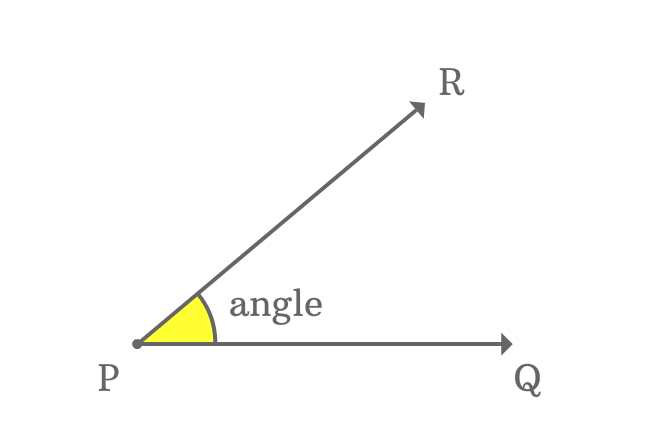# Vertex of an angle

A point where an angle is made by the intersection of two lines, is called vertex of an angle.

## Example$\overrightarrow{PQ}$ and $\overrightarrow{PR}$ are two lines and they both meet each other at their common point $P$.

The intersection of the rays $\overrightarrow{PQ}$ and $\overrightarrow{PR}$ formed an angle at point $P$. The point is the only reason for forming the angle between them. Therefore, the point $P$ is called vertex of the angle.

So, every point where an angle is made by the intersection of the lines, is known as vertex of the angle.

Latest Math Topics
Jun 26, 2023
Jun 23, 2023

###### Math Questions

The math problems with solutions to learn how to solve a problem.

Learn solutions

Practice now

###### Math Videos

The math videos tutorials with visual graphics to learn every concept.

Watch now

###### Subscribe us

Get the latest math updates from the Math Doubts by subscribing us.